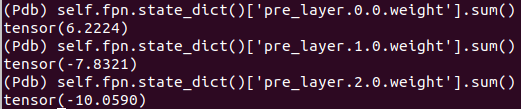# nn.ModuleList() seems not to support * operation?

I found that if I construct one ModuleList by this way:

``````self.pre_layer = nn.ModuleList([nn.Sequential(nn.Conv2d(256, 256, kernel_size=3, padding=1),
nn.ReLU(inplace=True))] * 3)
``````

This weights of the three Conv2d are always keeping the same.But their ids are different:What’s the reason?
Besides, if use for loop to construct a ModuleList, then everything works well.

``````self.pre_layer = nn.ModuleList([nn.Sequential(nn.Conv2d(256, 256, kernel_size=3, padding=1),
nn.ReLU(inplace=True)) for i in range(3)])
``````